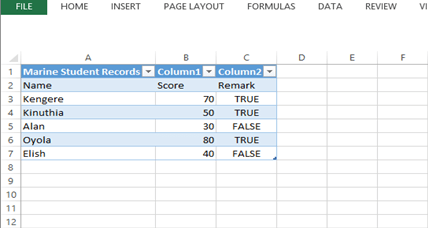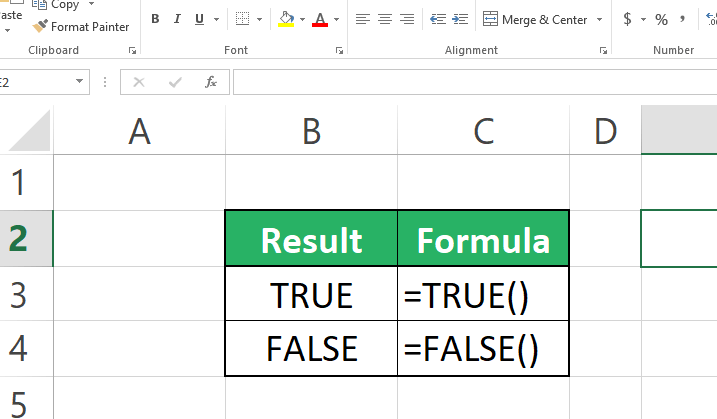# How to use true and false functions in Excel

Microsoft Excel is an excellent tool often used to keep track of data and other useful records. Among the various capabilities entailed in excel is the use of function and not only so but those that are conditional by nature. This conditional function operates under the same principle of Boolean logic. In our case, instead of 1's and O's, we have True and False. This type of formulae is very powerful in its simplicity.

For example, you might be a class teacher. You may need to calculate and see the student's performance in relation to the mean score.

Having noticed the importance of the true and false, it is time to dive into its use!!!

## How to use the true and false function in Microsoft Excel

1. Have a list of data in place to compare.. as in my case, the data is belowIn our case, with the data provided, we will be checking those that have attained the mean mark, which is 50.

For those who have attained 50 and above, the remark would display true, and those that are 49 and below False will be what we expect.

2. In cell C3, write the conditional function If (B3>= 50, True(), False())In our table, since the first column B3, Kengere is above average, the outcome will be true3. Repeat the same process in the remaining cells## Method 2

Excel comes with inbuilt true and false functions. For example, if you type =TRUE() a TRUE value will be returned.

Alternatively if you type =FALSE() a false value will be returnedHowever, you don't have to use these functions under normal circumstances.  In the above table, TRUE represents 1 while False represents 0.

Knowing that we can apply it to make calculations. The formula multiplies the result of TRUE by 25 and vice versa## Conclusion

That was simple, is it so? Hoping the guide has been simple and useful as you notice how powerful a tool the true and false function is, and advancing your knowledge in its application will only make Excel desirable and easy to use